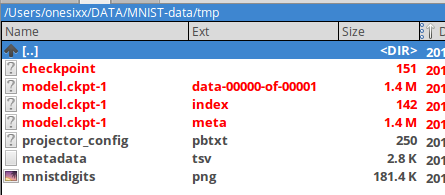# Simple Introduction to Tensorboard Embedding Visualisation

###### – gibhub:  https://github.com/rmeertens/Simplest-Tensorflow-Tensorboard-MNIST-Embedding-Visualisation/blob/master/Minimal%20example%20embeddings.ipynb

```### Visualising embeddings
# It helps you understand what your algorithm learned, and if this is what you expected it to learn
# Embedding visualisation is a standard feature in Tensorboard.
#
# the most simple code to get a simple visualisation of MNIST digits running.

%matplotlib inline
import matplotlib.pyplot as plt
import tensorflow as tf
import numpy as np
import os

from tensorflow.contrib.tensorboard.plugins import projector
from tensorflow.examples.tutorials.mnist import input_data

LOG_DIR = '/Users/onesixx/DATA/MNIST-data/tmp'
NAME_TO_VISUALISE_VARIABLE = "mnistembedding"
TO_EMBED_COUNT = 500

PATH_FOR_MNIST_SPRITES  =  os.path.join(LOG_DIR,'mnistdigits.png')

### What to visualise
# Although the embedding visualiser is meant for visualising embeddings obtained after training,
# you can also use it to apply visualisation of normal MNIST digits.
# In this case, each digit is represented by a vector with length 28*28=784 dimensions.

mnist = input_data.read_data_sets("MNIST_data/", one_hot=False)
batch_xs, batch_ys = mnist.train.next_batch(TO_EMBED_COUNT)```

sprite & metadata 준비

```#Visualisation helper functions

# the sprites
# If you don’t load sprites, each digit is represented as a simple point (does NOT give you a lot of information)
# To add labels, you have to create a ‘sprite map’ basically all images in what you want to visualise…

########################################################################
# There are 3 functions which are quite important for the visualisation.

### create_sprite_image:
# neatly aligns image sprits on a square canvas, as specified in the images section here:
# (https://www.tensorflow.org/get_started/embedding_viz)

def create_sprite_image(images):
"""Returns a sprite image consisting of images passed as argument. Images should be count x width x height"""
if isinstance(images, list):
images = np.array(images)
img_h = images.shape
img_w = images.shape
n_plots = int(np.ceil(np.sqrt(images.shape)))

spriteimage = np.ones((img_h * n_plots ,img_w * n_plots ))

for i in range(n_plots):
for j in range(n_plots):
this_filter = i * n_plots + j
if this_filter < images.shape:
this_img = images[this_filter]
spriteimage[i * img_h:(i + 1) * img_h,
j * img_w:(j + 1) * img_w] = this_img
return spriteimage

### vector_to_matrix_mnist:
# MNIST characters are loaded as a vector, not as an image… this function turns them into images

def vector_to_matrix_mnist(mnist_digits):
"""Reshapes normal mnist digit (batch,28*28) to matrix (batch,28,28)"""
return np.reshape(mnist_digits,(-1,28,28))

### invert_grayscale:
# matplotlib treats a 0 as black, and a 1 as white.
# The tensorboard embeddings visualisation looks way better with white backgrounds,
# so we invert them for the visualisation

def invert_grayscale(mnist_digits):
""" Makes black white, and white black """
return 1-mnist_digits

### Save the sprite image
# Pretty straightforward:
# convert our vectors to images, invert the grayscale, and create and save the sprite image.
to_visualise = batch_xs
to_visualise = vector_to_matrix_mnist(to_visualise)
to_visualise = invert_grayscale(to_visualise)

sprite_image = create_sprite_image(to_visualise)

plt.imsave(PATH_FOR_MNIST_SPRITES,sprite_image,cmap='gray')
plt.imshow(sprite_image,cmap='gray')

### Save the metadata
# To add colors to your mnist digits,
# the embedding visualisation tool needs to know what label each image has.
# This is saved in a “TSV (tab seperated file)”.

# Each line of our file contains the following:
# "Index" , "Label"
# The Index is simply the index in our embedding matrix.
# The label is the label of the MNIST character.

# This code writes our data to the metadata file.
with open(PATH_FOR_MNIST_METADATA,'w') as f:
f.write("Index\tLabel\n")
for index,label in enumerate(batch_ys):
f.write("%d\t%d\n" % (index,label))
```

```### Creating the embeddings
# For this example the embeddings are extremely simple:
# they are the direct values of the traindata.
# Your graph will probably be more complicated,
# but the important thing is that you know the name of the variable you want to visualise

embedding_var = tf.Variable(batch_xs, name=NAME_TO_VISUALISE_VARIABLE)
summary_writer = tf.summary.FileWriter(LOG_DIR)

### Create the embedding projector
# This is the important part of your embedding visualisation.
# Here you specify what variable you want to project, what the metadata path is (the names and classes),
# and where you save the sprites.

config = projector.ProjectorConfig()
embedding.tensor_name = embedding_var.name

# Specify where you find the metadata

# Specify where you find the sprite (we will create this later)
embedding.sprite.image_path = PATH_FOR_MNIST_SPRITES #'mnistdigits.png'
embedding.sprite.single_image_dim.extend([28,28])

# Say that you want to visualise the embeddings => /Users/onesixx/Dropbox/Rhome/tensorflow/projector_config.pbtxt
projector.visualize_embeddings(summary_writer, config)

### Saving the data
# Tensorboard loads the saved variable from the saved graph.
# Initialise a session and variables, and
# save them in your logging directory.
# 'tmp/model.ckpt-1'

sess = tf.InteractiveSession()
sess.run(tf.global_variables_initializer())
saver = tf.train.Saver()
saver.save(sess, os.path.join(LOG_DIR, "model.ckpt"), 1)

# How to run
tensorboard –logdir=tmp
```Categories: DL

Blog Owner